We get started with our exercise by collecting data. The data is generated by a function unknown to you. This precondition makes this example more realistic, as in real applications we do not know the exact specifications of the underlying data generation process either. At the end of this section we will uncover the secret of the data generation process.

This is the data, our observations, in tabular form. We have 25 data points, each data point is a $$(x,y)$$ pair.

poly_data
##             x           y
## 1  0.28757752  1.16443996
## 2  0.78830514 -0.88780200
## 3  0.40897692  0.17413059
## 4  0.88301740 -0.21743732
## 5  0.94046728 -0.07645417
## 6  0.04555650  0.26285704
## 7  0.52810549 -0.45020937
## 8  0.89241904 -0.88236568
## 9  0.55143501 -0.39313237
## 10 0.45661474  0.15201462
## 11 0.95683335 -0.64790568
## 12 0.45333416  0.25912870
## 13 0.67757064 -0.52350905
## 14 0.57263340 -0.49157948
## 15 0.10292468  0.19461123
## 16 0.89982497 -0.87515511
## 17 0.24608773  1.23942552
## 18 0.04205953  0.14918285
## 19 0.32792072  0.42349275
## 20 0.95450365 -0.49184749
## 21 0.88953932 -0.68494536
## 22 0.69280341 -0.62575853
## 23 0.64050681 -0.82552774
## 24 0.99426978  0.07943064
## 25 0.65570580 -1.95912808

Here is the data in form of a scatter plot:

plot(poly_data$x, poly_data$y)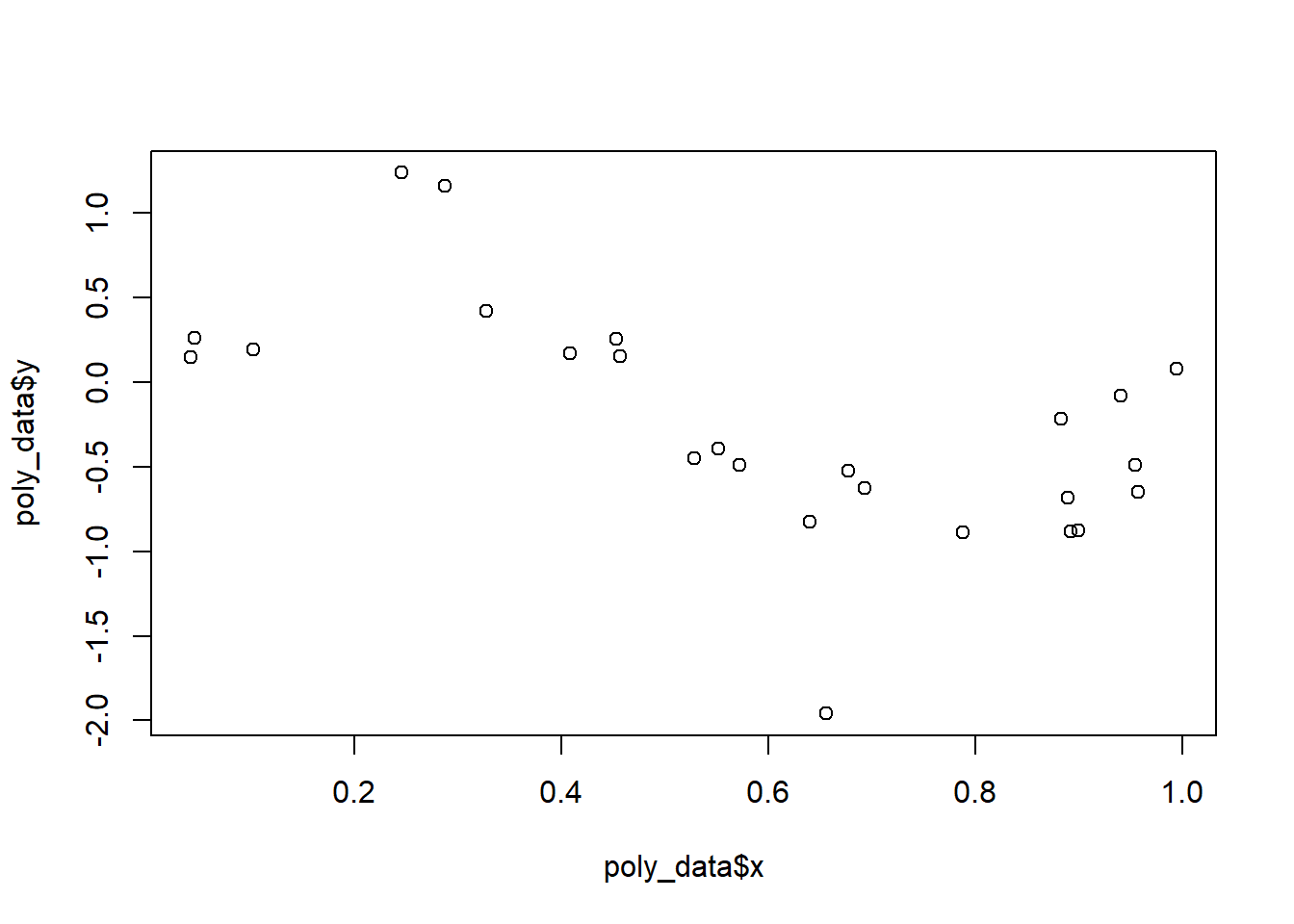#### Fitting a curve in R: The notation

The statistical software R provides powerful functionality to fit a polynomial to data. One of these functions is the lm() function, which we already know from simple linear regression. However, in order to fit a $$k^{th}$$-order polynomial we need to add additional arguments to the function call. In addition, there are two different options of coding a polynomial regression.

For a 3rd-order polynomial the first option is to type lm(response ~ poly(predictor, 3)) and the second option is lm(response ~ predictor + I(predictor^2) + I(predictor^3))). We need the I(...) notation to prevent any special interpretation of operators in the model formula.

The difference between those two options is, that in the first case the poly() function produces orthogonal polynomials, which have the advantage that the inspection of the regression coefficient allows to assess which polynomial significantly improved the regression. However, in this case the numerical values for $$\beta_i$$ may no longer be plugged into the polynomial regression equation from above. In contrast, if we apply the second option, the resulting regression coefficients may be plugged into the regression equation and yield the correct numerical result. However, as the predictors are correlated (problem of multicollinearity) it is often difficult to identify which polynomial significantly improved the regression.

Note: The prediction itself will not be affected at all by the choice of notation.

To alleviate the confusion we will calculate an example in R. For this we construct two 2nd-order polynomials for poly_data:

m1 <- lm(y ~ poly(x, 2), data = poly_data)
m2 <- lm(y ~ x + I(x^2), data = poly_data)

We review the output of both models:

m1
##
## Call:
## lm(formula = y ~ poly(x, 2), data = poly_data)
##
## Coefficients:
## (Intercept)  poly(x, 2)1  poly(x, 2)2
##     -0.2374      -1.9608       0.5614
m2
##
## Call:
## lm(formula = y ~ x + I(x^2), data = poly_data)
##
## Coefficients:
## (Intercept)            x       I(x^2)
##      0.8325      -2.8237       1.3861

As noted above, the regression coefficients differ. Let us predict $$\hat y$$ for $$x=0.5$$ by applying the predict() function:

predict(m1, newdata = list("x" = 0.5))
##          1
## -0.2328107
predict(m2, newdata = list("x" = 0.5))
##          1
## -0.2328107

As expected, both models predict $$\hat y = -0.2328107$$ for $$x=0.5$$.

#### Fitting a curve in R (continued)

So now that we know the notation in R we start to build 6 different models, with $$k = 1,2,3,5,9,14$$. For each model we calculate the RMSE. Finally, we plot the data together with the regression line of each particular model. For convenience we construct a loop to reduce the amount of coding.

# plotting setup
par(mfrow = c(3, 2), mar = c(2, 2, 1, 1)) # set up 6 subplots

# setup
RMSE <- data.frame("kth_order" = NA, "RMSE" = NA) # empty data frame to store RMSE
vals <- list("x" <- seq(min(poly_data$x), max(poly_data$y), by = 0.01)) # set up vector used for prediction

# run  loop
k <- c(1, 2, 3, 5, 9, 14) # k-th order

for (i in 1:length(k)) {
# build models
model <- lm(y ~ poly(x, k[i]), data = poly_data)

# calculate RMSE and store it for further usage
RMSE[i, 1] <- k[i] # store k-th order
RMSE[i, 2] <- sqrt(sum((fitted(model) - poly_data$y)^2) / length(poly_data$y)) # calculate RMSE

# predict
predictions <- predict(model, newdata = vals)
# plot
plot(poly_data$x, poly_data$y, pch = 16, col = "blue")
lines(vals[], predictions, lwd = 2, col = "red")
text(x = 0.8, y = 0.95, paste0("k = ", k[i], ", RMSE = ", round(RMSE[i, 2], 3))) # annotate the plot
}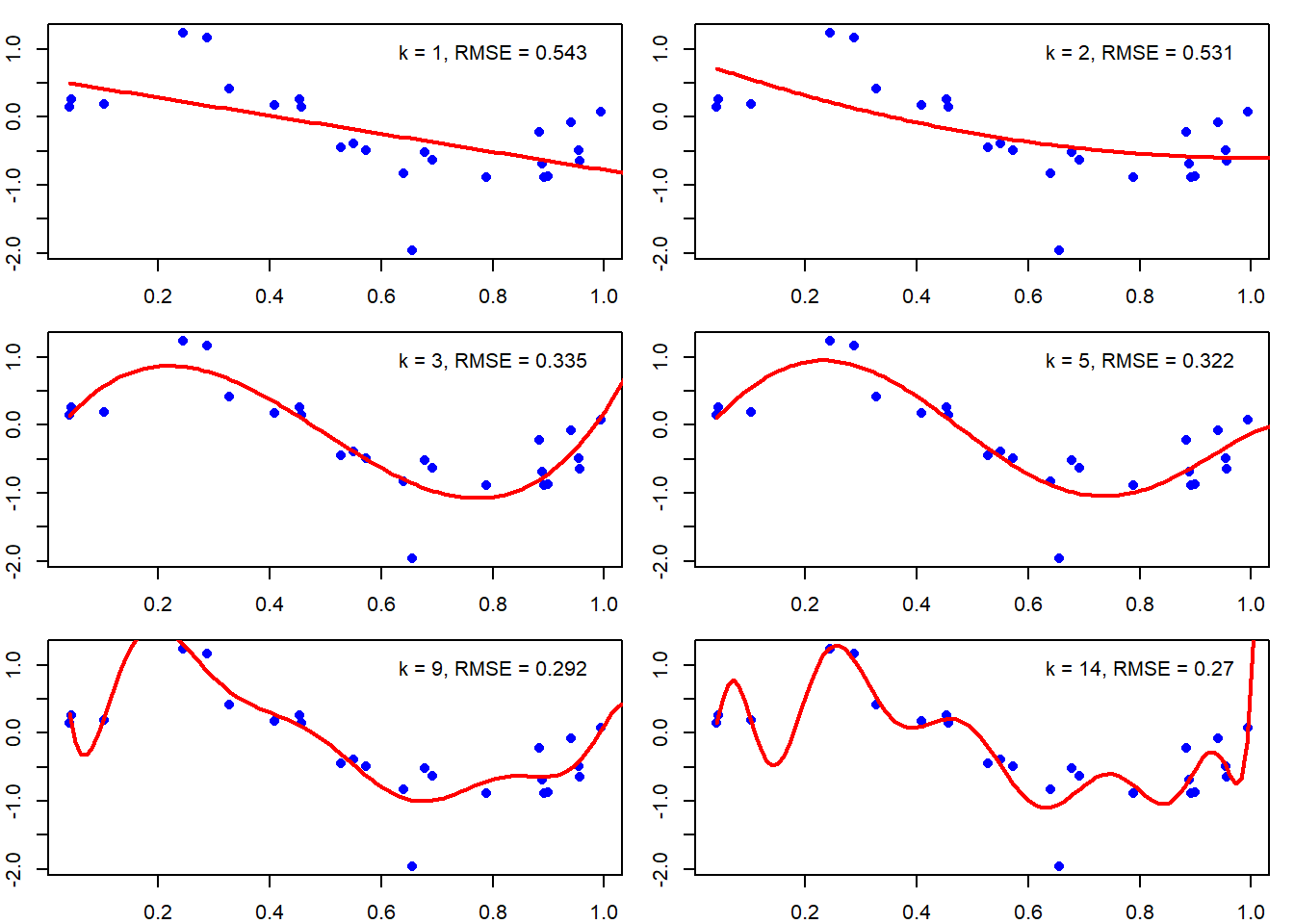Awesome, pretty plots! The figure shows, that if we increase $$k$$, the order of the polynomial, the curve becomes more flexible and it fits the data better and better. The better the data is fitted, the lower becomes the error (RMSE).

In order to visualize that behavior we plot the RMSE against $$k$$:

plot(RMSE[, 1], RMSE[, 2],
xlab = "k-th order",
ylab = "RMSE",
type = "b",
col = "blue",
pch = 16
)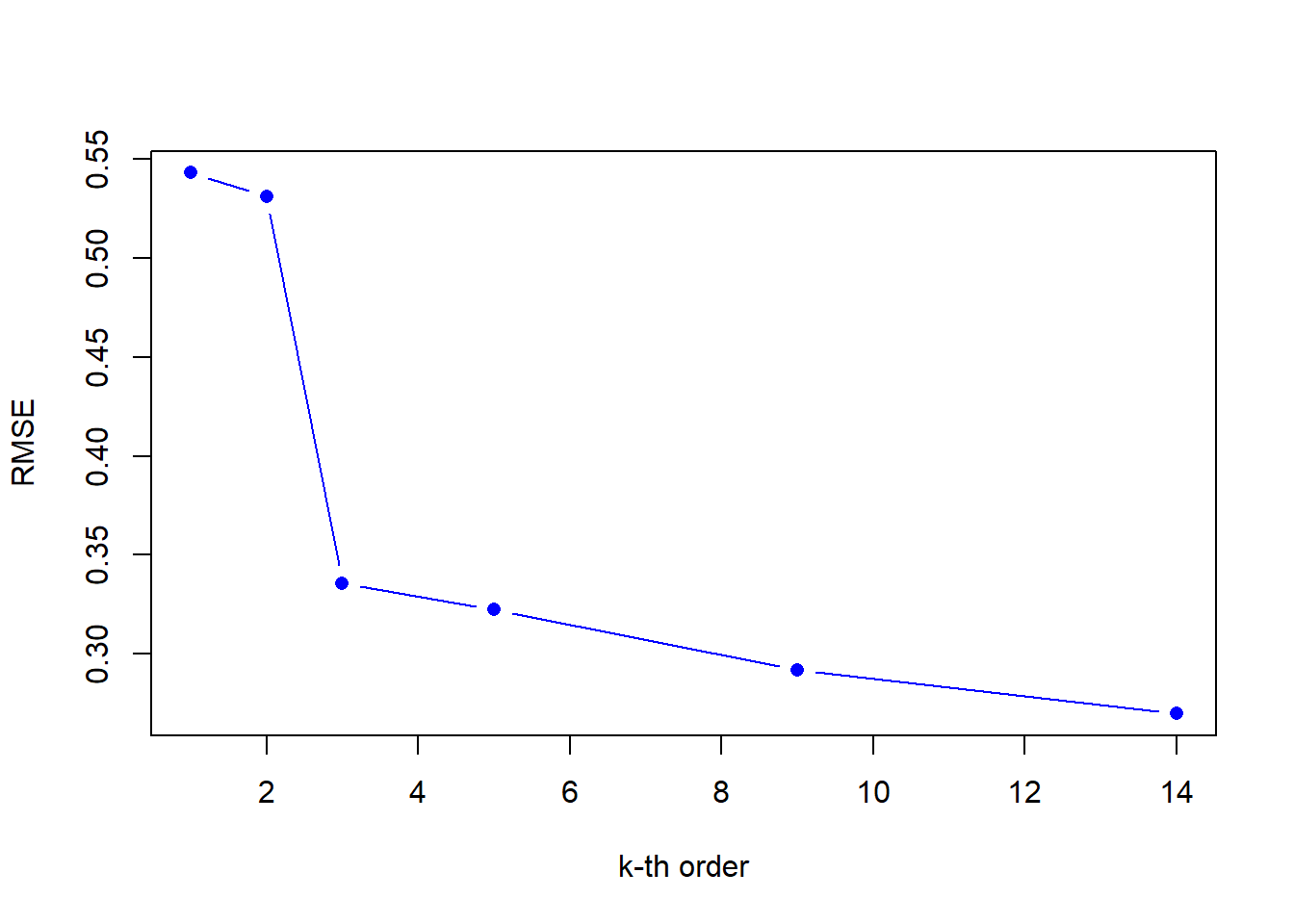Hence, once again the question arises: which is the best polynomial to fit the data? Do we believe that the 14th-order polynomial fits the underlying data generation process best? Even though we obtain an excellent fit to the observation data by increasing the order of the polynomial, it remains questionable if the high order polynomial generalizes well. Imagine we conduct a new measurement campaign and receive new data based on the same data generation process. Do you believe the wildly oscillating curve of a high order polynomial will still fit the data well? No, probably not!

This problem is known as overfitting. Recall, the goal is to learn the model parameters from the data. Thus, we are interested in achieving a good generalization of the model and not necessarily perfectly fitted observation data.

#### Learning from Data

So, how do we solve the problem? How do we determine the best $$k^{th}$$-order polynomial for our data set? Well, there are many methods and strategies to counteract overfitting. In this section we follow a simple approach. First, we split the data set into two parts. We call one part the training set and the other part the validation set. Then we use all the data in the training set to learn the model parameters $$\beta_i$$, in the same fashion as we did above. Thereafter, we apply the trained model to predict the data of the validation set and evaluate the model performance by computing the RMSE. Thus, we use the validation set to optimize the model’s complexity, given by $$k$$.

Unfortunately, if we want to learn from data we ultimately need data to learn from. So far we worked with 25 observations. That is not much. In real life applications we would probably have to obtain new observations by conducting a new measurement campaign. In our exercise, however, we may generate more data fairly easily. Thus, we continue this example with a new data set of 150 observations.

Let us plot the data!

plot(new_poly_data$x, new_poly_data$y)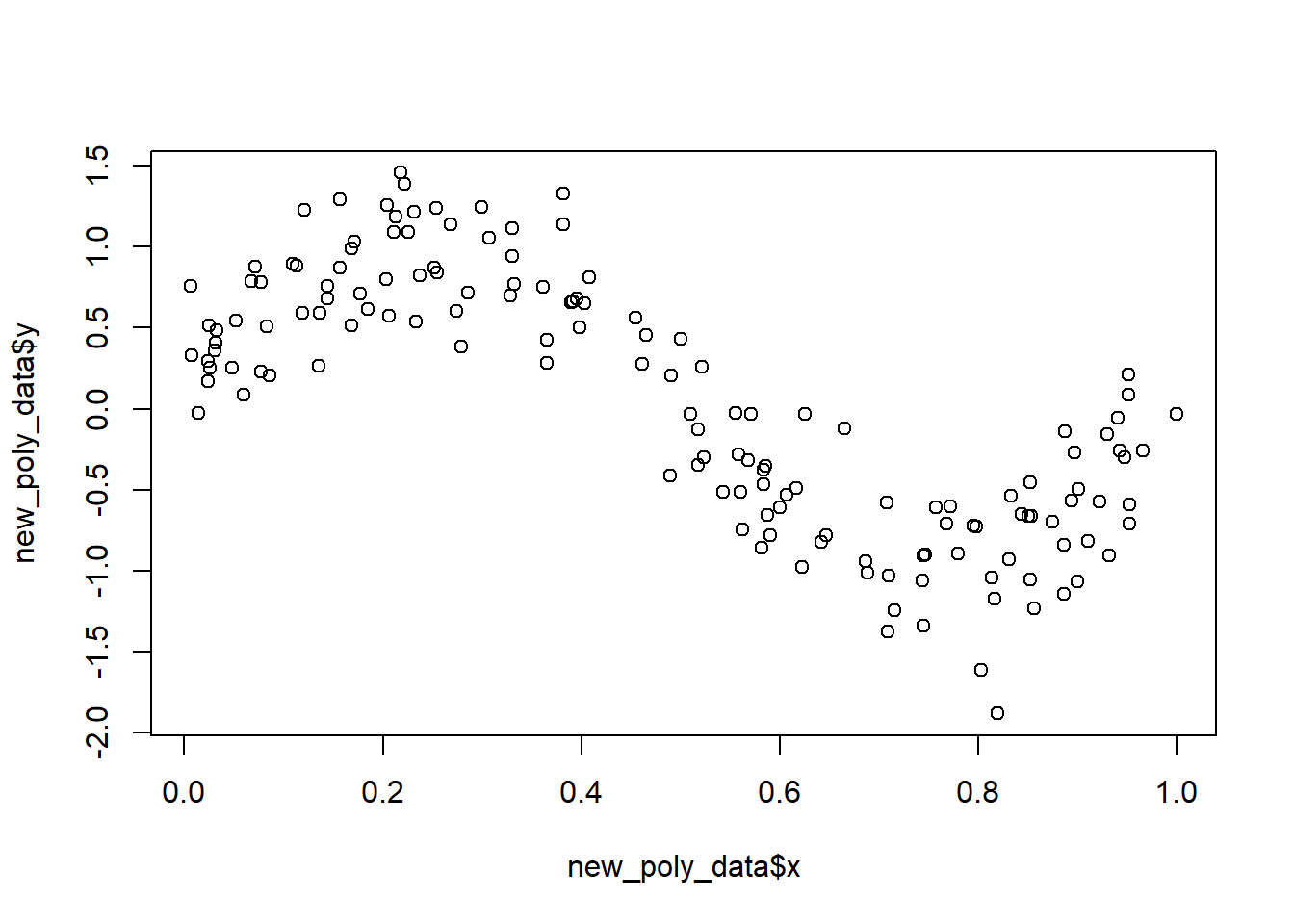##### Training set and validation set

Now, we are ready to build our training and validation set. For this we use the sample.split() function from the caTools package. We split the data in such a way, that 65 % of the data is assigned to the training set and the rest, 35 % of the data, is assigned to the validation set.

library(caTools)
set.seed(100) # set seed for reproducibility

# define split vector
split <- sample.split(new_poly_data$y, SplitRatio = 0.65) # split data set train_set <- new_poly_data[split == TRUE, ] val_set <- new_poly_data[split == FALSE, ] # check dimensions of the training and validation set dim(train_set) ##  97 2 dim(val_set) ##  53 2 ##### Model building and model evaluation In this exercise we are going to build 14 models with $$k = 1,2,...,14$$. We evaluate each of the 14 polynomial regression models by calculating the RMSE on the training set. Further, after building the model and obtaining the model parameters $$\beta_i$$, we use the model to predict the response variable in the validation set. Again, we rely on the RMSE in order to evaluate the predictions based on the validation set. Finally, we plot the RMSE of each model for both, the training set and the validation set. Based on the RMSE we assess the generalization of the model. We already developed a nice piece of code above. Thus, we only modify and extend the existing code: # setup RMSE <- data.frame("kth_order" = NA, "RMSE_train" = NA, "RMSE_val" = NA) # empty data frame to store RMSE vals <- list("x" <- seq(min(train_set$x), max(train_set$y), by = 0.01)) # set up vector used for prediction # run loop k <- seq(1, 14) # k-th order for (i in k) { # build models model <- lm(y ~ poly(x, k[i]), data = train_set) # calculate RMSE and store it for further usage RMSE[i, 1] <- k[i] # store k-th order RMSE[i, 2] <- sqrt(sum((fitted(model) - train_set$y)^2) / length(train_set$y)) # calculate RMSE of the training set # predict predictions <- predict(model, newdata = val_set) RMSE[i, 3] <- sqrt(sum((predictions - val_set$y)^2) / length(val_set$y)) # calculate RMSE of the validation set } # plot RMSE for training and validation set plot(RMSE[, 1], RMSE[, 2], xlab = "k-th order", ylab = "RMSE", ylim = c(min(RMSE[, c(2, 3)]), max(RMSE[, c(2, 3)])), type = "b", col = "blue", pch = 16 ) lines(RMSE[, 3], type = "b", col = "red", pch = 16 ) legend("topright", legend = c("training set", "validation set"), lty = c(1, 1), col = c("blue", "red") )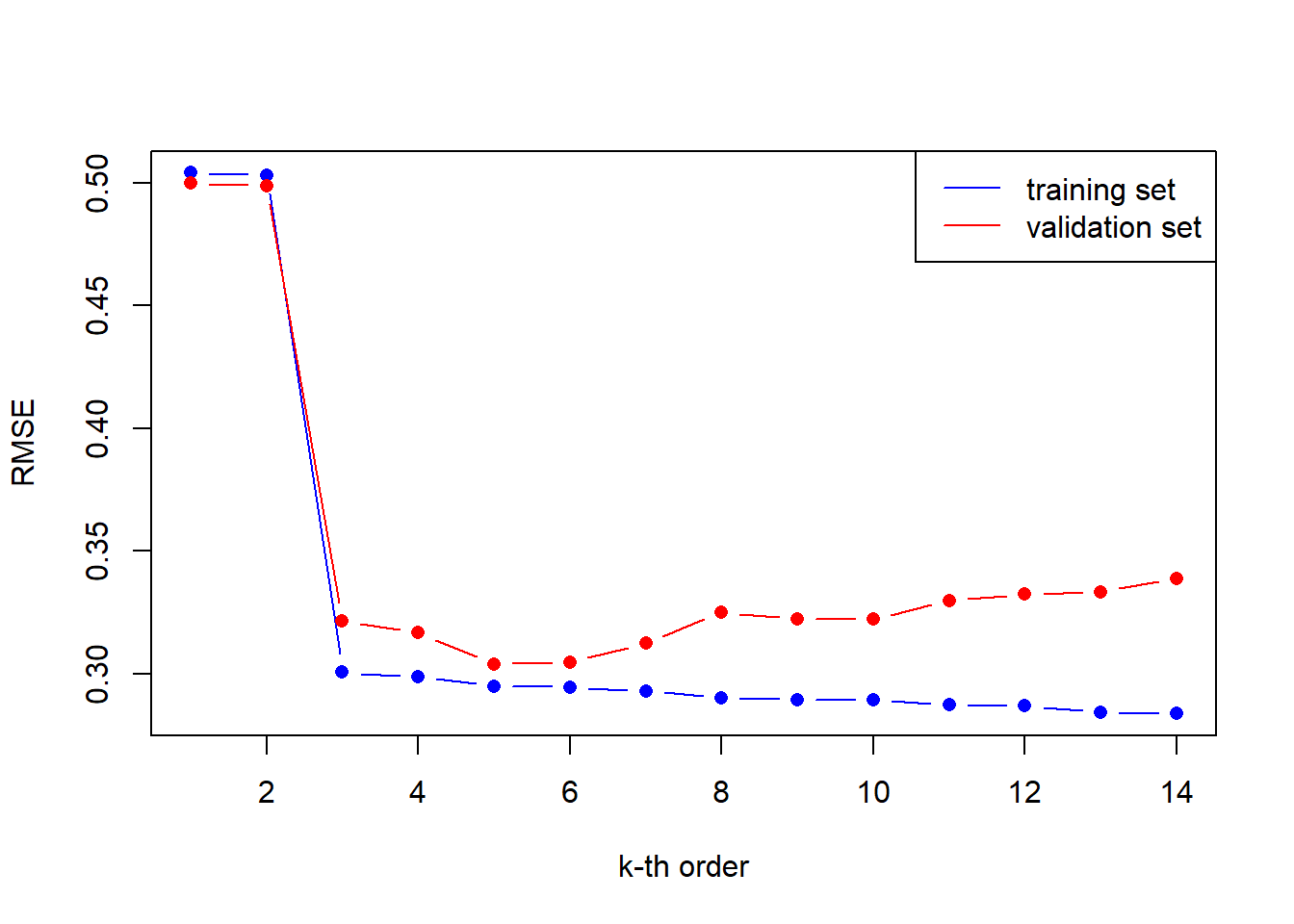The figure shows, that the error on the training data (blue line) is constantly decreasing. This makes perfect sense, the more complex the model becomes by increasing $$k$$, the better the model fits the training data. We observed the same behavior in the section above, when we trained our model with just 25 observations. If we take a look at the RMSE for the validation set (red line), we see that with increasing $$k$$, and thus increasing model complexity, the error decreases. However, there is a sweet spot, indicated by the lowest RMSE, where the model is just complex enough to generalize well on the so far unseen validation data. If the model complexity increases further, the RMSE is starting to increase, too. This indicates that the model is overfitting. Thus, the model memorizes the data of the training set well, however, the model becomes less predictive for so far unseen data, such as the data of the validation set. Take a look at the figure above to see that the lowest error on the validation set is obtained for a regression model of 5th-order. ##### Model presentation In the previous section we found a 5th-order polynomial regression model to perform best on the validation set. Now, we plot that model, including the 95%-confidence intervals, on the data set in order to visually assess its quality. In addition, we plot the underlying function for the data generation process. best_order <- RMSE[which.min(RMSE[, 3]), 1] final_model <- lm(y ~ poly(x, best_order), data = train_set) # predictions vals <- list("x" <- seq(min(new_poly_data$x), max(new_poly_data$y), by = 0.01)) predictions <- predict(final_model, newdata = vals, interval = "confidence", level = 0.95) # plot data plot(new_poly_data$x, new_poly_data\$y, pch = 16, ylab = "", xlab = "")

# plot data generation function
lines(vals[], sin(2 * pi * vals[]), lwd = 3, col = "green")

# plot fit and confidence levels
lines(vals[], predictions[, "fit"], lwd = 2, col = "blue")
lines(vals[], predictions[, "upr"], lwd = 2, lty = 2, col = "blue")
lines(vals[], predictions[, "lwr"], lwd = 2, lty = 2, col = "blue")

legend("topright",
legend = c("observed data", "prediction", "signal"),
lty = c(NA, 1, 1),
pch = c(16, NA, NA),
cex = 0.75,
col = c(1, "blue", "green"),
lwd = c(NA, 2, 2)
)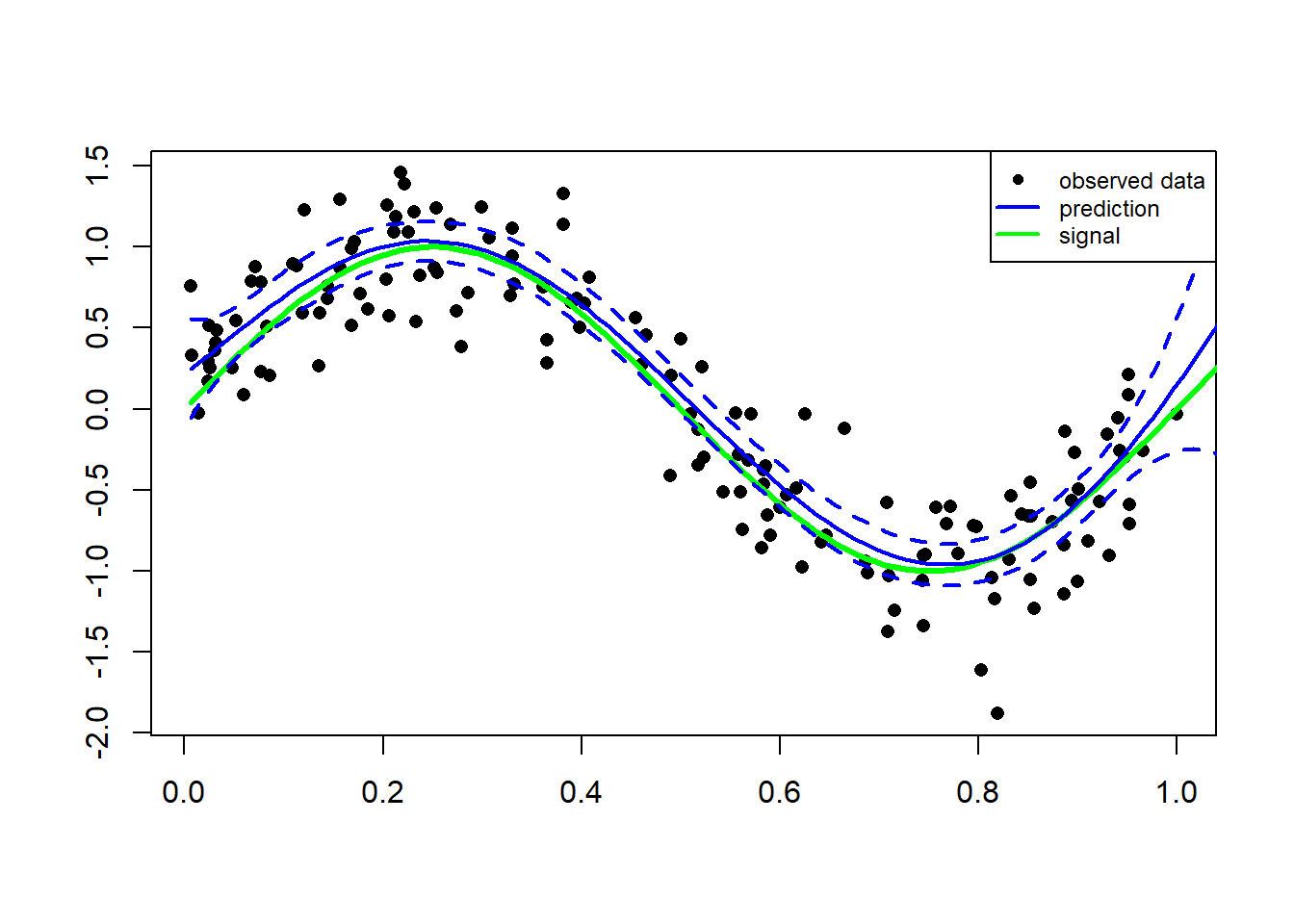The figure shows that our model does a decent job at fitting the data and therefore we can be quite satisfied with it.

#### Data generating function

The input values $$x_n$$ for the underlying function are generated uniformly in range $$U(0, 1)$$. The corresponding target values $$y$$ are obtained by first computing the corresponding values of the function $$sin(2\pi x)$$ and then adding random noise with a Gaussian distribution with a standard deviation of $$0.35$$.

##### Data generating function #####
set.seed(123) # set seed for reproducibility
n <- 25
x <- runif(n, 0, 1)
y <- sin(2 * pi * x) + rnorm(n, 0, 0.35)
poly_data <- data.frame("x" = x, "y" = y)

Citation

The E-Learning project SOGA-R was developed at the Department of Earth Sciences by Kai Hartmann, Joachim Krois and Annette Rudolph. You can reach us via mail by soga[at]zedat.fu-berlin.de.You may use this project freely under the Creative Commons Attribution-ShareAlike 4.0 International License.

Please cite as follow: Hartmann, K., Krois, J., Rudolph, A. (2023): Statistics and Geodata Analysis using R (SOGA-R). Department of Earth Sciences, Freie Universitaet Berlin.